# A quick sort algorithm to sort Vectors or arrays. Provides sort and binary search capabilities. : Sort Search « Collections Data Structure « Java

A quick sort algorithm to sort Vectors or arrays. Provides sort and binary search capabilities.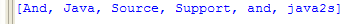```
// HTMLParser Library - A java-based parser for HTML
// http://htmlparser.org
// Copyright (C) 2006 Derrick Oswald
//
// Revision Control Information
//
// \$URL: https://svn.sourceforge.net/svnroot/htmlparser/trunk/lexer/src/main/java/org/htmlparser/util/sort/Sort.java \$
// \$Author: derrickoswald \$
// \$Date: 2006-09-16 10:44:17 -0400 (Sat, 16 Sep 2006) \$
// \$Revision: 4 \$
//
// This library is free software; you can redistribute it and/or
// modify it under the terms of the Common Public License; either
// version 1.0 of the License, or (at your option) any later version.
//
// This library is distributed in the hope that it will be useful,
// but WITHOUT ANY WARRANTY; without even the implied warranty of
// MERCHANTABILITY or FITNESS FOR A PARTICULAR PURPOSE. See the
// Common Public License for more details.
//
// You should have received a copy of the Common Public License
// along with this library; if not, the license is available from
// the Open Source Initiative (OSI) website:

//package org.htmlparser.util.sort;

import java.util.Enumeration;
import java.util.Hashtable;
import java.util.Vector;

/**
* A quick sort algorithm to sort Vectors or arrays. Provides sort and binary
* search capabilities.
* <p>
* This all goes away in JDK 1.2.
* <p>
*
* @author James Gosling
* @author Kevin A. Smith
* @author Derrick Oswald
* @version 1.4, 11 June, 1997
*/
public class Sort {
/**
* No object of this class need ever be instantiated. All methods are
* static.
*/
private Sort() {
}

/**
* This is a generic version of C.A.R Hoare's Quick Sort algorithm. This
* will handle vectors that are already sorted, and vectors with duplicate
* keys. Equivalent to:
*
* <pre>
* QuickSort(v, 0, v.size() - 1);
* </pre>
*
* @param v
*            A <code>Vector</code> of <code>Ordered</code> items.
* @exception ClassCastException
*                If the vector contains objects that are not
*                <code>Ordered</code>.
*/
public static void QuickSort(Vector v) throws ClassCastException {
QuickSort(v, 0, v.size() - 1);
}

/**
* This is a generic version of C.A.R Hoare's Quick Sort algorithm. This
* will handle vectors that are already sorted, and vectors with duplicate
* keys.
* <p>
* If you think of a one dimensional vector as going from the lowest index
* on the left to the highest index on the right then the parameters to this
* function are lowest index or left and highest index or right.
*
* @param v
*            A <code>Vector</code> of <code>Ordered</code> items.
* @param lo0
*            Left boundary of vector partition.
* @param hi0
*            Right boundary of vector partition.
* @exception ClassCastException
*                If the vector contains objects that are not
*                <code>Ordered</code>.
*/
public static void QuickSort(Vector v, int lo0, int hi0)
throws ClassCastException {
int lo = lo0;
int hi = hi0;
Ordered mid;

if (hi0 > lo0) { // arbitrarily establish partition element as the
// midpoint of the vector
mid = (Ordered) v.elementAt((lo0 + hi0) / 2);

// loop through the vector until indices cross
while (lo <= hi) {
// find the first element that is greater than or equal to
// the partition element starting from the left index
while ((lo < hi0)
&& (0 > ((Ordered) v.elementAt(lo)).compare(mid)))
++lo;

// find an element that is smaller than or equal to
// the partition element starting from the right index
while ((hi > lo0)
&& (0 < ((Ordered) v.elementAt(hi)).compare(mid)))
--hi;

// if the indexes have not crossed, swap
if (lo <= hi)
swap(v, lo++, hi--);
}

// if the right index has not reached the left side of array
// must now sort the left partition
if (lo0 < hi)
QuickSort(v, lo0, hi);

// if the left index has not reached the right side of array
// must now sort the right partition
if (lo < hi0)
QuickSort(v, lo, hi0);
}
}

private static void swap(Vector v, int i, int j) {
Object o;

o = v.elementAt(i);
v.setElementAt(v.elementAt(j), i);
v.setElementAt(o, j);
}

/**
* This is a generic version of C.A.R Hoare's Quick Sort algorithm. This
* will handle arrays that are already sorted, and arrays with duplicate
* keys.
* <p>
* Equivalent to:
*
* <pre>
* QuickSort(a, 0, a.length - 1);
* </pre>
*
* @param a
*            An array of <code>Ordered</code> items.
*/
public static void QuickSort(Ordered[] a) {
QuickSort(a, 0, a.length - 1);
}

/**
* This is a generic version of C.A.R Hoare's Quick Sort algorithm. This
* will handle arrays that are already sorted, and arrays with duplicate
* keys.
* <p>
* If you think of a one dimensional array as going from the lowest index on
* the left to the highest index on the right then the parameters to this
* function are lowest index or left and highest index or right.
*
* @param a
*            An array of <code>Ordered</code> items.
* @param lo0
*            Left boundary of array partition.
* @param hi0
*            Right boundary of array partition.
*/
public static void QuickSort(Ordered[] a, int lo0, int hi0) {
int lo = lo0;
int hi = hi0;
Ordered mid;

if (hi0 > lo0) {
// arbitrarily establish partition element as the midpoint of the
// array
mid = a[(lo0 + hi0) / 2];

// loop through the vector until indices cross
while (lo <= hi) {
// find the first element that is greater than or equal to
// the partition element starting from the left index
while ((lo < hi0) && (0 > a[lo].compare(mid)))
++lo;

// find an element that is smaller than or equal to
// the partition element starting from the right Index.
while ((hi > lo0) && (0 < a[hi].compare(mid)))
--hi;

// if the indexes have not crossed, swap
if (lo <= hi)
swap(a, lo++, hi--);
}

// if the right index has not reached the left side of array
// must now sort the left partition
if (lo0 < hi)
QuickSort(a, lo0, hi);

// if the left index has not reached the right side of array
// must now sort the right partition
if (lo < hi0)
QuickSort(a, lo, hi0);
}
}

/**
* Swaps two elements of an array.
*
* @param a
*            The array of elements.
* @param i
*            The index of one item to swap.
* @param j
*            The index of the other item to swap.
*/
private static void swap(Object[] a, int i, int j) {
Object o;
o = a[i];
a[i] = a[j];
a[j] = o;
}

/**
* This is a string version of C.A.R Hoare's Quick Sort algorithm. This will
* handle arrays that are already sorted, and arrays with duplicate keys.
* <p>
* Equivalent to:
*
* <pre>
* QuickSort(a, 0, a.length - 1);
* </pre>
*
* @param a
*            An array of <code>String</code> items.
*/
public static void QuickSort(String[] a) {
QuickSort(a, 0, a.length - 1);
}

/**
* This is a string version of C.A.R Hoare's Quick Sort algorithm. This will
* handle arrays that are already sorted, and arrays with duplicate keys.
* <p>
* If you think of a one dimensional array as going from the lowest index on
* the left to the highest index on the right then the parameters to this
* function are lowest index or left and highest index or right.
*
* @param a
*            An array of <code>String</code> items.
* @param lo0
*            Left boundary of array partition.
* @param hi0
*            Right boundary of array partition.
*/
public static void QuickSort(String[] a, int lo0, int hi0) {
int lo = lo0;
int hi = hi0;
String mid;

if (hi0 > lo0) {
// arbitrarily establish partition element as the midpoint of the
// array
mid = a[(lo0 + hi0) / 2];

// loop through the vector until indices cross
while (lo <= hi) {
// find the first element that is greater than or equal to
// the partition element starting from the left index
while ((lo < hi0) && (0 > a[lo].compareTo(mid)))
++lo;

// find an element that is smaller than or equal to
// the partition element starting from the right Index.
while ((hi > lo0) && (0 < a[hi].compareTo(mid)))
--hi;

// if the indexes have not crossed, swap
if (lo <= hi)
swap(a, lo++, hi--);
}

// if the right index has not reached the left side of array
// must now sort the left partition
if (lo0 < hi)
QuickSort(a, lo0, hi);

// if the left index has not reached the right side of array
// must now sort the right partition
if (lo < hi0)
QuickSort(a, lo, hi0);
}
}

/**
* This is a generic version of C.A.R Hoare's Quick Sort algorithm. This
* will handle Sortable objects that are already sorted, and Sortable
* objects with duplicate keys.
* <p>
*
* @param sortable
*            A <code>Sortable</code> object.
* @param lo0
*            Left boundary of partition.
* @param hi0
*            Right boundary of partition.
*/
public static void QuickSort(Sortable sortable, int lo0, int hi0) {
int lo = lo0;
int hi = hi0;
Ordered mid;
Ordered test;

if (hi0 > lo0) { // arbitrarily establish partition element as the
// midpoint of the vector
mid = sortable.fetch((lo0 + hi0) / 2, null);
test = null;

// loop through the vector until indices cross
while (lo <= hi) {
// find the first element that is greater than or equal to
// the partition element starting from the left index
while ((lo < hi0)
&& (0 > (test = sortable.fetch(lo, test)).compare(mid)))
++lo;

// find an element that is smaller than or equal to
// the partition element starting from the right index
while ((hi > lo0)
&& (0 < (test = sortable.fetch(hi, test)).compare(mid)))
--hi;

// if the indexes have not crossed, swap
if (lo <= hi)
sortable.swap(lo++, hi--);
}

// if the right index has not reached the left side of array
// must now sort the left partition
if (lo0 < hi)
QuickSort(sortable, lo0, hi);

// if the left index has not reached the right side of array
// must now sort the right partition
if (lo < hi0)
QuickSort(sortable, lo, hi0);
}
}

/**
* This is a generic version of C.A.R Hoare's Quick Sort algorithm. This
* will handle Sortable objects that are already sorted, and Sortable
* objects with duplicate keys.
* <p>
* Equivalent to:
*
* <pre>
* QuickSort(sortable, sortable.first(), sortable.last());
* </pre>
*
* @param sortable
*            A <code>Sortable</code> object.
*/
public static void QuickSort(Sortable sortable) {
QuickSort(sortable, sortable.first(), sortable.last());
}

/**
* Sort a Hashtable.
*
* @param h
*            A Hashtable with String or Ordered keys.
* @return A sorted array of the keys.
* @exception ClassCastException
*                If the keys of the hashtable are not <code>Ordered</code>.
*/
public static Object[] QuickSort(Hashtable h) throws ClassCastException {
Enumeration e;
boolean are_strings;
Object[] ret;

// make the array
ret = new Ordered[h.size()];
e = h.keys();
are_strings = true; // until proven otherwise
for (int i = 0; i < ret.length; i++) {
ret[i] = e.nextElement();
if (are_strings && !(ret[i] instanceof String))
are_strings = false;
}

// sort it
if (are_strings)
QuickSort((String[]) ret);
else
QuickSort((Ordered[]) ret);

return (ret);
}

/**
* Binary search for an object
*
* @param set
*            The collection of <code>Ordered</code> objects.
* @param ref
*            The name to search for.
* @param lo
*            The lower index within which to look.
* @param hi
*            The upper index within which to look.
* @return The index at which reference was found or is to be inserted.
*/
public static int bsearch(Sortable set, Ordered ref, int lo, int hi) {
int num;
int mid;
Ordered ordered;
int half;
int result;
int ret;

ret = -1;

num = (hi - lo) + 1;
ordered = null;
while ((-1 == ret) && (lo <= hi)) {
half = num / 2;
mid = lo + ((0 != (num & 1)) ? half : half - 1);
ordered = set.fetch(mid, ordered);
result = ref.compare(ordered);
if (0 == result)
ret = mid;
else if (0 > result) {
hi = mid - 1;
num = ((0 != (num & 1)) ? half : half - 1);
} else {
lo = mid + 1;
num = half;
}
}
if (-1 == ret)
ret = lo;

return (ret);
}

/**
* Binary search for an object
*
* @param set
*            The collection of <code>Ordered</code> objects.
* @param ref
*            The name to search for.
* @return The index at which reference was found or is to be inserted.
*/
public static int bsearch(Sortable set, Ordered ref) {
return (bsearch(set, ref, set.first(), set.last()));
}

/**
* Binary search for an object
*
* @param vector
*            The vector of <code>Ordered</code> objects.
* @param ref
*            The name to search for.
* @param lo
*            The lower index within which to look.
* @param hi
*            The upper index within which to look.
* @return The index at which reference was found or is to be inserted.
*/
public static int bsearch(Vector vector, Ordered ref, int lo, int hi) {
int num;
int mid;
int half;
int result;
int ret;

ret = -1;

num = (hi - lo) + 1;
while ((-1 == ret) && (lo <= hi)) {
half = num / 2;
mid = lo + ((0 != (num & 1)) ? half : half - 1);
result = ref.compare(vector.elementAt(mid));
if (0 == result)
ret = mid;
else if (0 > result) {
hi = mid - 1;
num = ((0 != (num & 1)) ? half : half - 1);
} else {
lo = mid + 1;
num = half;
}
}
if (-1 == ret)
ret = lo;

return (ret);
}

/**
* Binary search for an object
*
* @param vector
*            The vector of <code>Ordered</code> objects.
* @param ref
*            The name to search for.
* @return The index at which reference was found or is to be inserted.
*/
public static int bsearch(Vector vector, Ordered ref) {
return (bsearch(vector, ref, 0, vector.size() - 1));
}

/**
* Binary search for an object
*
* @param array
*            The array of <code>Ordered</code> objects.
* @param ref
*            The name to search for.
* @param lo
*            The lower index within which to look.
* @param hi
*            The upper index within which to look.
* @return The index at which reference was found or is to be inserted.
*/
public static int bsearch(Ordered[] array, Ordered ref, int lo, int hi) {
int num;
int mid;
int half;
int result;
int ret;

ret = -1;

num = (hi - lo) + 1;
while ((-1 == ret) && (lo <= hi)) {
half = num / 2;
mid = lo + ((0 != (num & 1)) ? half : half - 1);
result = ref.compare(array[mid]);
if (0 == result)
ret = mid;
else if (0 > result) {
hi = mid - 1;
num = ((0 != (num & 1)) ? half : half - 1);
} else {
lo = mid + 1;
num = half;
}
}
if (-1 == ret)
ret = lo;

return (ret);
}

/**
* Binary search for an object
*
* @param array
*            The array of <code>Ordered</code> objects.
* @param ref
*            The name to search for.
* @return The index at which reference was found or is to be inserted.
*/
public static int bsearch(Ordered[] array, Ordered ref) {
return (bsearch(array, ref, 0, array.length - 1));
}
}

// HTMLParser Library - A java-based parser for HTML
// http://htmlparser.org
// Copyright (C) 2006 Derrick Oswald
//
// Revision Control Information
//
// \$URL:
// https://svn.sourceforge.net/svnroot/htmlparser/trunk/lexer/src/main/java/org/htmlparser/util/sort/Ordered.java
// \$
// \$Author: derrickoswald \$
// \$Date: 2006-09-16 10:44:17 -0400 (Sat, 16 Sep 2006) \$
// \$Revision: 4 \$
//
// This library is free software; you can redistribute it and/or
// modify it under the terms of the Common Public License; either
// version 1.0 of the License, or (at your option) any later version.
//
// This library is distributed in the hope that it will be useful,
// but WITHOUT ANY WARRANTY; without even the implied warranty of
// MERCHANTABILITY or FITNESS FOR A PARTICULAR PURPOSE. See the
// Common Public License for more details.
//
// You should have received a copy of the Common Public License
// along with this library; if not, the license is available from
// the Open Source Initiative (OSI) website:

/**
* Describes an object that knows about ordering. Implementors must have a
* comparison function, which imposes a partial ordering on some collection of
* objects. Ordered objects can be passed to a sort method (such as
* org.htmlparser.util.sort.Sort) to allow precise control over the sort order.
* <p>
* An set of elements S is partially ordered if and only if
* <code>e1.compare(e2)==0</code> implies that <code>e1.equals(e2)</code> for
* every e1 and e2 in S.
* <p>
* This all goes away in JDK 1.2.
* <p>
* For use with java.lang.Comparable from JDK 1.2:
*
* <pre>
* public int compare(Object o1, Object o2) {
*   return (((Ordered) o1).compare(o2));
* }
* </pre>
*
* @see Sort
*/
interface Ordered {
/**
* Compares this object with another for order. Returns a negative integer,
* zero, or a positive integer as this object is less than, equal to, or
* greater than the second.
* <p>
* The implementor must ensure that
* <code>sgn(x.compare(y)) == -sgn(y.compare(x))</code> for all x and y.
* (This implies that <code>x.compare(y)</code> must throw an exception if
* and only if <code>y.compare(x)</code> throws an exception.)
* <p>
* The implementor must also ensure that the relation is transitive:
* <code>((x.compare(y)>0) && (y.compare(z)>0))</code> implies
* <code>x.compare(z)>0</code>.
* <p>
* Finally, the implementer must ensure that <code>x.compare(y)==0</code>
* implies that <code>sgn(x.compare(z))==sgn(y.compare(z))</code> for all z.
*
* @param that
*            The object to compare this object against.
* @return A negative integer, zero, or a positive integer as this object is
*         less than, equal to, or greater than the second.
* @exception ClassCastException
*                The arguments type prevents it from being compared by this
*                Ordered.
*/
public int compare(Object that);
}

// HTMLParser Library - A java-based parser for HTML
// http://htmlparser.org
// Copyright (C) 2006 Derrick Oswald
//
// Revision Control Information
//
// \$URL:
// https://svn.sourceforge.net/svnroot/htmlparser/trunk/lexer/src/main/java/org/htmlparser/util/sort/Sortable.java
// \$
// \$Author: derrickoswald \$
// \$Date: 2006-09-16 10:44:17 -0400 (Sat, 16 Sep 2006) \$
// \$Revision: 4 \$
//
// This library is free software; you can redistribute it and/or
// modify it under the terms of the Common Public License; either
// version 1.0 of the License, or (at your option) any later version.
//
// This library is distributed in the hope that it will be useful,
// but WITHOUT ANY WARRANTY; without even the implied warranty of
// MERCHANTABILITY or FITNESS FOR A PARTICULAR PURPOSE. See the
// Common Public License for more details.
//
// You should have received a copy of the Common Public License
// along with this library; if not, the license is available from
// the Open Source Initiative (OSI) website:

/**
* Provides a mechanism to abstract the sort process. Classes implementing this
* interface are collections of Ordered objects that are to be sorted by the
* Sort class and are not necessarily Vectors or Arrays of Ordered objects.
*
* @see Sort
*/
interface Sortable {
/**
* Returns the first index of the Sortable.
*
* @return The index of the first element.
*/
public int first();

/**
* Returns the last index of the Sortable.
*
* @return The index of the last element. If this were an array object this
*         would be (object.length - 1).
*/
public int last();

/**
* Fetch the object at the given index.
*
* @param index
*            The item number to get.
* @param reuse
*            If this argument is not null, it is an object acquired from a
*            previous fetch that is no longer needed and may be returned as
*            the result if it makes mores sense to alter and return it than
*            to fetch or create a new element. That is, the reuse object is
*            garbage and may be used to avoid allocating a new object if
*            that would normally be the strategy.
* @return The Ordered object at that index.
*/
public Ordered fetch(int index, Ordered reuse);

/**
* Swaps the elements at the given indicies.
*
* @param i
*            One index.
* @param j
*            The other index.
*/
public void swap(int i, int j);
}

```

### Related examples in the same category

 1 Linear search 2 Animation for quick sort 3 Quick Sort Implementation with median-of-three partitioning and cutoff for small arrays 4 Simple Sort Demo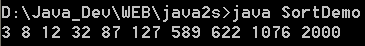5 A simple applet class to demonstrate a sort algorithm 6 Sorting an array of Strings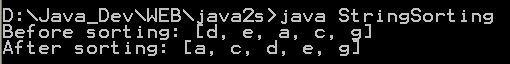7 Simple version of quick sort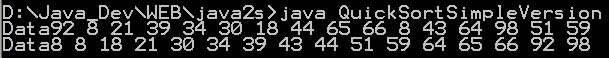8 Combine Quick Sort Insertion Sort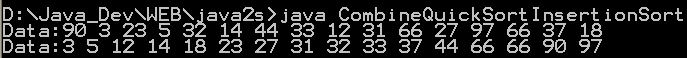9 Quick sort with median-of-three partitioning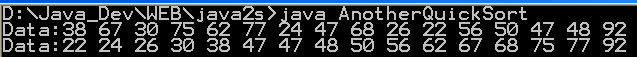10 Fast Quick Sort 11 Selection sort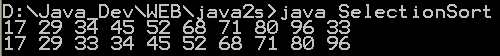12 Insert Sort for objects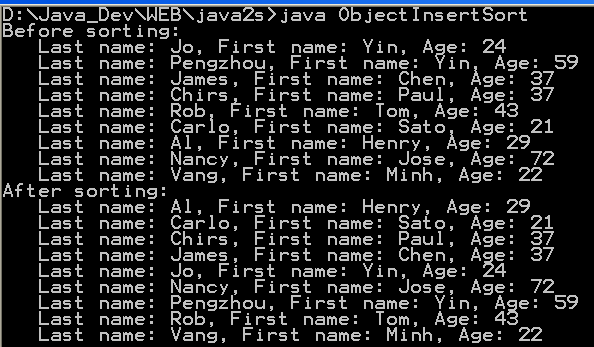13 Insert sort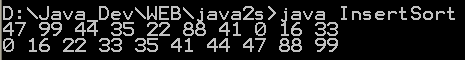14 Bubble sort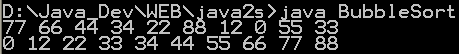15 Merge sort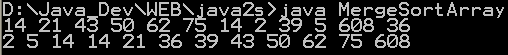16 Fast Merge Sort 17 Binary Search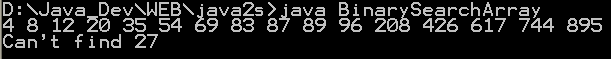18 Shell sort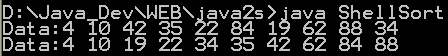19 Recursive Binary Search Implementation in Java 20 Topological sorting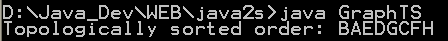21 Heap sort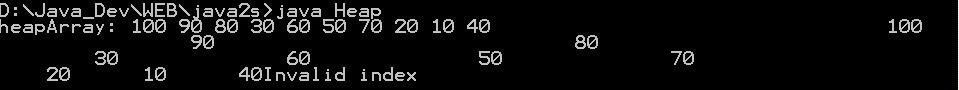22 Sort Numbers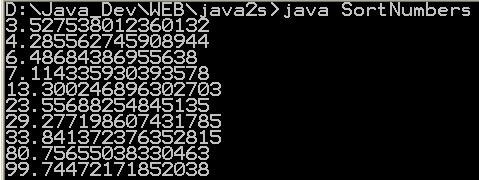23 A quick sort demonstration algorithm24 Performing Binary Search on Java byte Array Example 25 Performing Binary Search on Java char Array Example 26 Performing Binary Search on Java double Array Example 27 Performing Binary Search on Java float Array Example 28 Performing Binary Search on Java int Array Example 29 Performing Binary Search on Java long Array Example 30 Performing Binary Search on Java short Array 31 Sort items of an array 32 Sort an array of objects 33 Sort a String array 34 Sort string array with Collator 35 Binary search routines 36 FastQ Sorts the [l,r] partition (inclusive) of the specfied array of Rows, using the comparator.37 A binary search implementation. 38 Handles QuickSort and all of its methods. 39 Implements QuickSort three different ways 40 Returns an array of indices indicating the order the data should be sorted in.